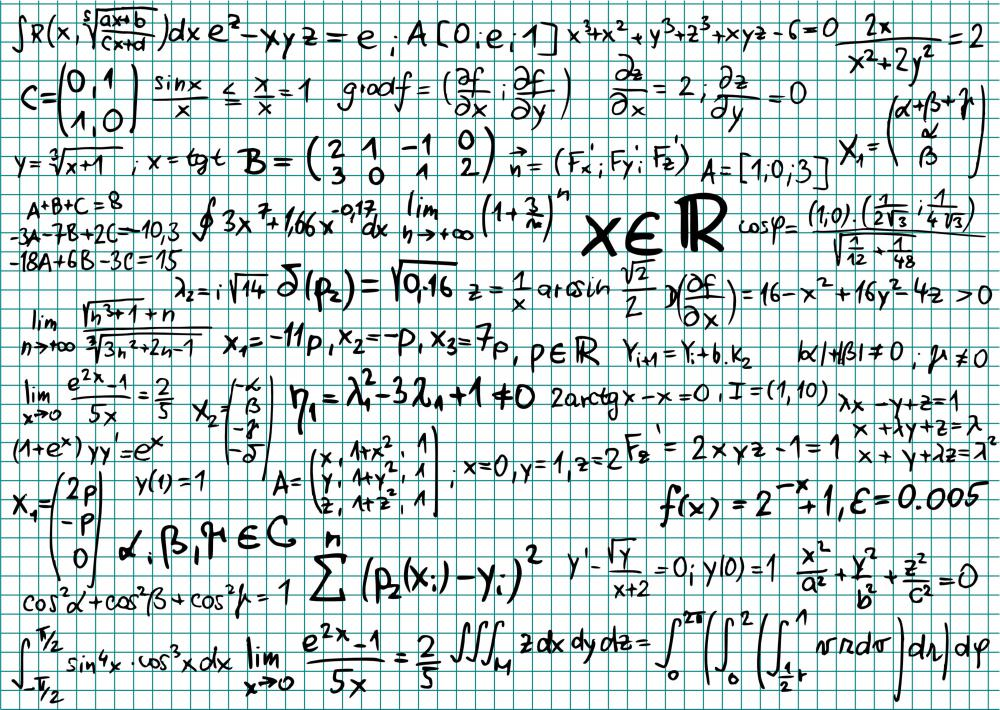# What Is Modern Econometrics?

Jim B.

Modern econometrics is the use of mathematics and statistics as a way to analyze economic phenomena and predict future outcomes. This is often done through the use of complex econometric models that portray the cause and effect of past or current economic stimuli. Econometric analysts can plug new data into these models as a means of predicting future results. One of the distinguishing features of modern econometrics is the use of complex computer algorithms that can crunch tremendous amounts of raw data and create a concise and coherent overview of some aspect of the economy.

For a long period of time in the past, economists could make hypotheses and guesses about the economy but couldn’t prove their theories without some sort of obvious sea change in the economy as an indicator. As a result, many started to use mathematics and statistics to give proof about their different ideas. Some began to realize that these same tools could actually give accurate assessments about future economic events, which is how the field of modern econometrics first came into being.Financial analysts use formulas to see what the potential damage might be from hypothetical economic catastrophes.

Although it can be defined in many different ways, modern econometrics essentially boils down to plugging statistical information about an economy into mathematical formulas. When that happens, the results can show cause and effect about certain economic characteristics. For example, when interest rates rise, it might affect employment levels, inflation, economic growth, and so on. Using econometrics, an analyst might be able to pinpoint exactly how and to what extent this occurs.

Economic models are a huge part of the field of modern econometrics. This is where the leaps and bounds made by computer technology in the modern era come into play. Sophisticated programs devised by analysts can take all of the information that is entered, analyze the relationships between the numerical data, and come up with specific information about how certain economic stimuli affect the overall picture. It is an effective way for those practicing econometrics to use the past to predict the future.

Proponents of modern econometrics should factor in those unforeseen circumstances that can trigger huge negative changes in an economy. One way to do this is to simulate worst-case scenarios for an economy. By doing this, analysts can see what the potential damage done by hypothetical economic catastrophes might be. In addition, models can be used to show the ways out of such dire occurrences. The boundaries for econometrics are practically limitless, but using them can be fruitless without sound economic theories as their basis.

## You might also Like